# Largest possible cone

It is necessary to make the largest possible cone from an iron rod in the shape of a prism with dimensions of 5.6 cm, 4.8 cm, 7.2 cm. a) Calculate its volume. b) Calculate the waste.

V =  43.4294 cm3
x =  150.1066 cm3

### Step-by-step explanation: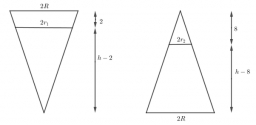Did you find an error or inaccuracy? Feel free to write us. Thank you!Tips to related online calculators
Tip: Our volume units converter will help you with the conversion of volume units.

#### You need to know the following knowledge to solve this word math problem:

We encourage you to watch this tutorial video on this math problem:

## Related math problems and questions:

• Max - cone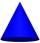From the iron bar (shape = prism) with dimensions 6.2 cm, 10 cm, 6.2 cm must be produced the greatest cone. a) Calculate cone volume. b) Calculate the waste.
• Square prismCalculate the volume of a square prism of high 2 dm wherein the base is: rectangle with sides 17 cm and 1.3 dm
• Largest wallFind the content of the largest wall of a prism with a rectangle base with a height of 4 dm, side c = 5 cm, and side b = 6 cm.
• Uboid volumeCalculate the cuboid volume if the walls are 30cm², 35cm², 42cm²
• Cuboid - edgesThe cuboid has dimensions in ratio 4: 3: 5, the shortest edge is 12 cm long. Find: (A) the lengths of the remaining edges, (B) the surface of the cuboid, (C) the volume of the cuboid
• ConeCircular cone of height 15 cm and volume 5699 cm3 is at one-third of the height (measured from the bottom) cut by a plane parallel to the base. Calculate the radius and circumference of the circular cut.The surface of the regular quadrilateral prism is 8800 cm2, the base edge is 20 cm long. Calculate the volume of the prism
• Body diagonalCalculate the volume of a cuboid whose body diagonal u is equal to 6.1 cm. Rectangular base has dimensions of 3.2 cm and 2.4 cm
• Prism - boxThe base of prism is a rectangle with a side of 7.5 cm and 12.5 cm diagonal. The volume of the prism is V = 0.9 dm3. Calculate the surface of the prism.
• Iron pole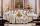What is the mass of a pole with the shape of a regular quadrilateral prism with a length of 1 m and a cross-sectional side length of a = 4.5 cm make from iron with density ρ = 7800 kg/m³?
• Cone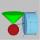Into rotating cone with dimensions r = 8 cm and h = 8 cm incribe cylinder with maximum volume so that the cylinder axis is perpendicular to the axis of the cone. Determine the dimensions of the cylinder.
• Mr. Gardener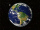Mr. Gardener wants to make wood for the balcony. Boxes. Each will have the shape of a perpendicular prism with a square base, the height is limited to 60 cm. Each container will be filled with soil by pouring the whole bag of substrate sold in a package w
• Sphere and coneWithin the sphere of radius G = 33 cm inscribe the cone with the largest volume. What is that volume, and what are the dimensions of the cone?
• Cuboid easyThe cuboid has the dimensions a = 12 cm, b = 9 cm, c = 36 cm. Calculate the length of the body diagonal of the cuboid.
• PresentGift box has a rectangular shape with dimensions of 8×8×3 cm. Miloslav wants to cover with square paper with sides of 18 cm. How much paper left him?
• Triangular prismCalculate the volume of a triangular prism 10 cm high, the base of which is an equilateral triangle with dimensions a = 5 cm and height va = 4,3 cm
• Pentagonal prismThe regular pentagonal prism is 10 cm high. The radius of the circle of the described base is 8 cm. Calculate the volume and surface area of the prism.